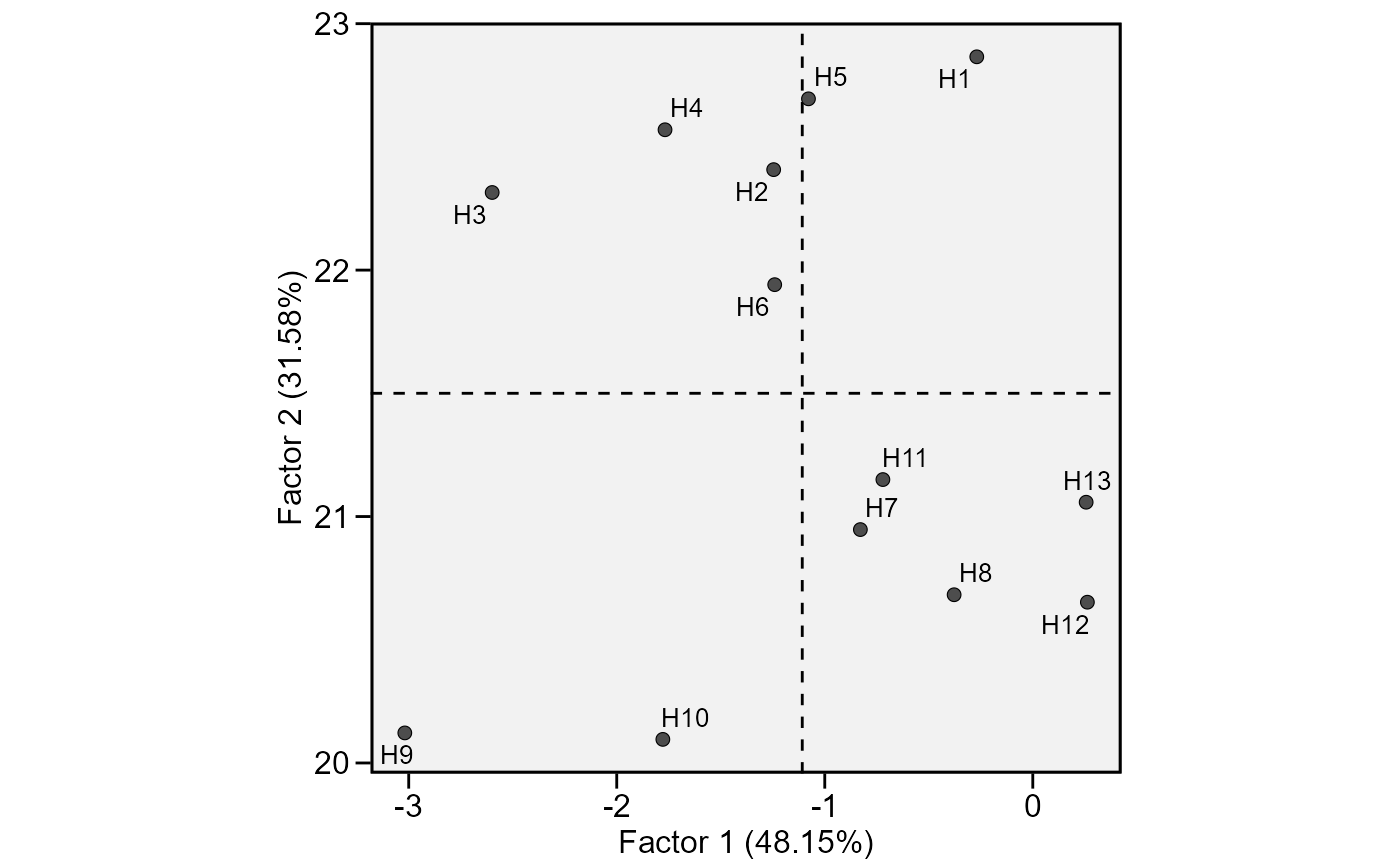This function plot the scores for genotypes obtained in the factor analysis to interpret the stability

## Usage

# S3 method for ge_factanal
plot(
x,
var = 1,
plot_theme = theme_metan(),
x.lim = NULL,
x.breaks = waiver(),
x.lab = NULL,
y.lim = NULL,
y.breaks = waiver(),
y.lab = NULL,
shape = 21,
col.shape = "gray30",
col.alpha = 1,
size.shape = 2.2,
size.bor.tick = 0.3,
size.tex.lab = 12,
size.tex.pa = 3.5,
force.repel = 1,
line.type = "dashed",
line.alpha = 1,
col.line = "black",
size.line = 0.5,
...
)

## Arguments

x

An object of class ge_factanal

var

The variable to plot. Defaults to var = 1 the first variable of x.

plot_theme

The graphical theme of the plot. Default is plot_theme = theme_metan(). For more details, see ggplot2::theme().

x.lim

The range of x-axis. Default is NULL (maximum and minimum values of the data set). New arguments can be inserted as x.lim = c(x.min, x.max).

x.breaks

The breaks to be plotted in the x-axis. Default is authomatic breaks. New arguments can be inserted as x.breaks = c(breaks)

x.lab

The label of x-axis. Each plot has a default value. New arguments can be inserted as x.lab = "my label".

y.lim

The range of x-axis. Default is NULL. The same arguments than x.lim can be used.

y.breaks

The breaks to be plotted in the x-axis. Default is authomatic breaks. The same arguments than x.breaks can be used.

y.lab

The label of y-axis. Each plot has a default value. New arguments can be inserted as y.lab = "my label".

shape

The shape for genotype indication in the plot. Default is 1 (circle). Values between 21-25: 21 (circle), 22 (square), 23 (diamond), 24 (up triangle), and 25 (low triangle) allows a color for fill the shape.

col.shape

The shape color for genotypes. Must be one value or a vector of colors with the same length of the number of genotypes. Default is "gray30". Other values can be attributed. For example, transparent_color(), will make a plot with only an outline around the shape area.

col.alpha

The alpha value for the color. Default is 1. Values must be between 0 (full transparency) to 1 (full color).

size.shape

The size of the shape (both for genotypes and environments). Default is 2.2.

size.bor.tick

The size of tick of shape. Default is 0.3. The size of the shape will be size.shape + size.bor.tick

size.tex.lab

The size of the text in the axes text and labels. Default is 12.

size.tex.pa

The size of the text of the plot area. Default is 3.5.

force.repel

Force of repulsion between overlapping text labels. Defaults to 1.

line.type

The type of the line that indicate the means in the biplot. Default is "solid". Other values that can be attributed are: "blank", no lines in the biplot, "dashed", "dotted", "dotdash", "longdash", and "twodash".

line.alpha

The alpha value that combine the line with the background to create the appearance of partial or full transparency. Default is 0.4. Values must be between "0" (full transparency) to "1" (full color).

col.line

The color of the line that indicate the means in the biplot. Default is "gray"

size.line

The size of the line that indicate the means in the biplot. Default is 0.5.

...

Currently not used..

## Value

An object of class gg, ggplot.

ge_factanal()

## Author

Tiago Olivoto tiagoolivoto@gmail.com

## Examples

# \donttest{
library(metan)
library(ggplot2)
model = ge_factanal(data_ge2,
env = ENV,
gen = GEN,
rep = REP,
resp = PH)
plot(model)plot(model,
size.shape = 3,
force.repel = 10,
col.shape = "orange",
col.line = "red")# }